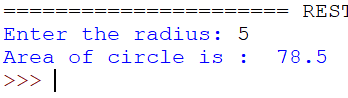# O Level Exam

#### Programming Example

WAP to print the area of circle when radius of the circle is given by user.

`Solution`

```r=int(input("Enter the radius: "))
area=3.14*r*r
print("Area of circle is : ",area)```
`Output`